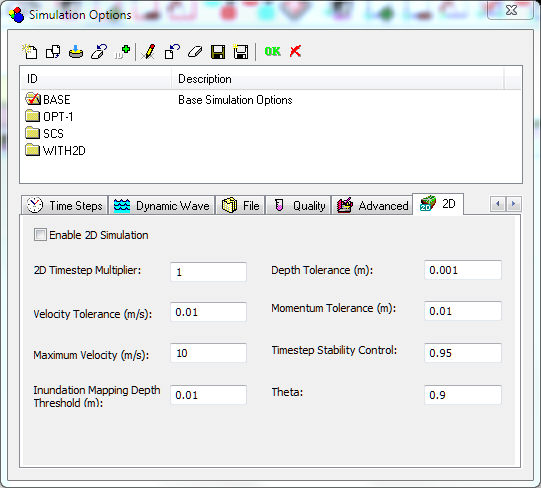#INFOSWMM

# 2D Simulation Options from InfoWorks ICM in InfoSWMM and InfoSWMM SA

If you have an InfoSWMM 2D license and you have set up your model with all of the necessary 2D elements and parameters, you can enable the 2D Simulation option. You must also set the 2D simulation options to fit the needs of your modeling scenario.Name Description Enable 2D Simulation Select this option to enable 2D simulation 2D Timestep Multiplier The 2D timestep should be a multiple (i.e. 1, 2, 3 etc.) of the routing timestep specified in Time Step Options. 2D Timestep = Routing Timestep * 2D Timestep Multiplier The calculations used by the 2D simulation are partially explicit and they are therefore influenced by the simulation time step. The recommended timestep will vary according to the size of the mesh. For relatively large meshes, a timestep of 20 – 30 seconds would be appropriate, but for smaller meshes, 5 seconds or even less should be used. The results should be checked for mass balance errors and if necessary the timestep reduced. Velocity Tolerance Mesh elements with water velocity below this value will have velocity reset to zero in terms of momentum calculations. Typical value: 0 Default value: 0 Maximum Velocity Velocity threshold limiting the velocities that can be achieved in an element in a 2D simulation. Mesh elements with water velocity above this value will have velocity reset to the maximum specified. Typical value: 33 ft/s (10 m/s) Inundation Mapping Depth Threshold Depth threshold used to determine Time to first inundation for mesh elements. Time to first inundation is reported as the time (from the start of the simulation) at which water depth in the mesh element first exceeds this threshold. Default value: 0.03 ft (0.01 m) Depth Tolerance The Depth Threshold is used to determine whether to consider a mesh element wet or dry. Mesh elements with depth of water below this value will be considered dry and a zero depth will be displayed in the output results. Only mass conservation will be considered in elements with depth below this threshold Typical value: 0.003 ft (0.001 m) Default value: 0.003 ft (0.001 m) Momentum Tolerance Depth threshold used to determine whether to consider momentum in a mesh element or not. Movement of water will not be calculated for mesh elements with depth of water below this value; only mass conservation will be taken into account. Typical value: 0.003-0.03 ft (0.001-0.01 m) Default value: 0.03 ft (0.01 m) Timestep Stability Control This parameter ensures that the internal timestep used by the 2D engine is within the stability bounds given by the CFL condition ( Courant Friedrichs LewyCourant R. Friedrichs K.O. and Lewy H. (1928) On the partial Difference Equations of Mathematical Physics. Math. Ann., Vol 100, p32. condition). Valid value: 0 < TSC < 1 Typical value: 0.95 Theta Weighting factor, θ, for the semi-implicit parameter. Weights the explicit and semi-implicit parts of the numerical scheme used for the time integration of the flow equations. The higher the value, the more weight given to the implicit part. Valid values: 0 < θ < 1 Typical value: 0.9